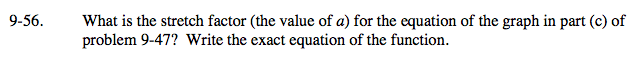### Home > A2C > Chapter Ch9 > Lesson 9.1.3 > Problem9-56

9-56.Using the equation that you found before, what value of y did you get for x = 0?
What do you need to multiply that by to get the appropriate value?

The stretch factor is 2.
The equation is y = −2(x + 2)2(x − 1).